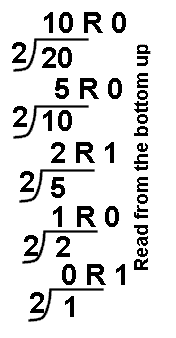Convert a number from any base to any other base:Convert 248 into base 10.   4 x 80 = 4   2 x 81 = 16 4 + 16 = 20 248 = 2010 And then convert that answer into base 2.2010 = 101002 Answer:  248 = 101002